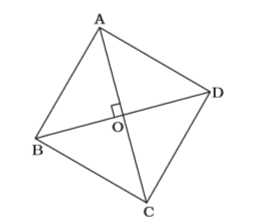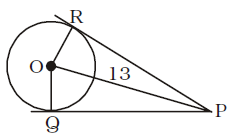## Mensuration

#### Mensuration

1. The radius of a circle is so increased that its circumference increases by 5%. The area of the circle then increases by:
1. 12.5%
2. 10.25%
3. 10.5%
4. None of these

1. Increase in radius of circle = Increase in circumference of circle = 5%

 ∴ Increase in area =5 + 5 + 5 × 5% = 10.25% 100

##### Correct Option: B

Increase in radius of circle = Increase in circumference of circle = 5%

 ∴ Increase in area =5 + 5 + 5 × 5% = 10.25% 100

1. The base of a right prism is a rightangled triangle whose sides are 5 cm, 12 cm and 13 cm. If the area of the total surface of the prism is 360 cm², then its height (in cm) is
1. 10
2. 12
3. 9
4. 11

1. Total surface area = Lateral surface area + 2 Area of base = Area of base × height + area of base

 ⇒ 360 = 30 × h + 1 × 5 × 12 2

⇒ 360 – 30 = 30 × h
⇒ 30h = 330
 ⇒ h = 300 = 10 cm. 30

##### Correct Option: A

Total surface area = Lateral surface area + 2 Area of base = Area of base × height + area of base

 ⇒ 360 = 30 × h + 1 × 5 × 12 2

⇒ 360 – 30 = 30 × h
⇒ 30h = 330
 ⇒ h = 300 = 10 cm. 30

1. The area of a rhombus is 216 cm² and the length of its one diagonal is 24 cm. The perimeter (in cm) of the rhombus is
1. 52
2. 60
3. 120
4. 100

1. Using Rule 12,Area of rhombus = 1 d1d2 2

 ⇒ 216 = 1 × 24 × d2 2

 ⇒ d2 = 216 = 18 12

∴ AO = 12 cm, BO = 9 cm
⇒ AB = √24² + 10² = √576 + 100
= √225 = 15 cm
∴ Perimeter of rhombus = 4 × 15 = 60 cm

##### Correct Option: B

Using Rule 12,Area of rhombus = 1 d1d2 2

 ⇒ 216 = 1 × 24 × d2 2

 ⇒ d2 = 216 = 18 12

∴ AO = 12 cm, BO = 9 cm
⇒ AB = √24² + 10² = √576 + 100
= √225 = 15 cm
∴ Perimeter of rhombus = 4 × 15 = 60 cm

1. The sides of a quadrilateral are in the ratio 3 : 4 : 5 : 6 and its perimeter is 72 cm. The length of its greatest side (in cm) is
1. 24
2. 27
3. 30
4. 36

1. 3x + 4x + 5x + 6x = 72
⇒ 18x = 72
⇒ x = 4
∴ Largest side = 6x = 6 × 4 = 24 cm.

##### Correct Option: A

3x + 4x + 5x + 6x = 72
⇒ 18x = 72
⇒ x = 4
∴ Largest side = 6x = 6 × 4 = 24 cm.

1. Point O is the centre of a circle of radius 5 cm. At a distance of 13 cm from O, a point P is taken. From this point, two tangents PQ and PR are drawn to the circle. Then , the area of quadrilateral PQOR is
1. 60 cm.²
2. 32.5 cm.²
3. 65 cm.²
4. 30 cm.²

1.OQ ⊥ QP ; OR ⊥ PR
PQ = PR = Tangents from anexterior point
OP is common.
∴ ∆ORP ≅ ∆OPQ
In right ∆OPQ,
OP = 13 cm., OQ = 5 cm.
∴ PQ = √13² - 5² = √169 - 25
= √144 = 12 cm.

 Area of ∆OPQ = 1 × 12 × 5 = 30 sq. cm. 2

∴ Area of quadrilateral PQOR = 2 × 30 = 60 sq. cm.

##### Correct Option: AOQ ⊥ QP ; OR ⊥ PR